# measures of central tendency.pptx

21 Dec 2022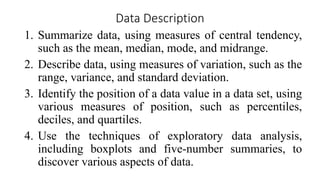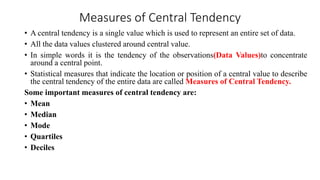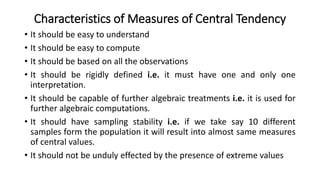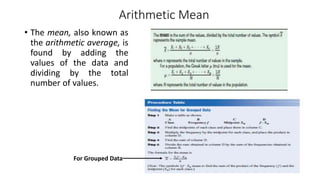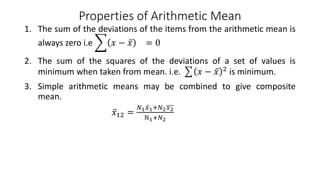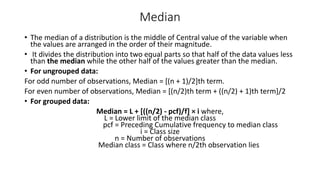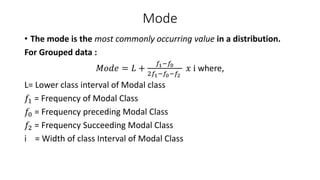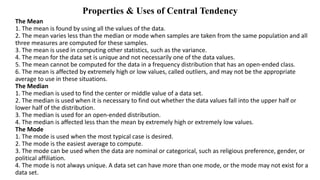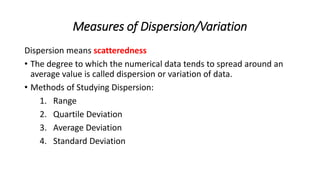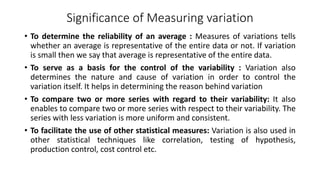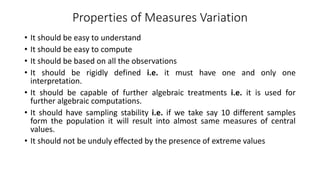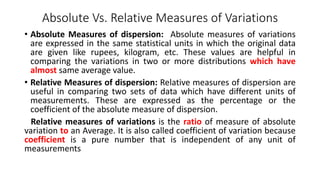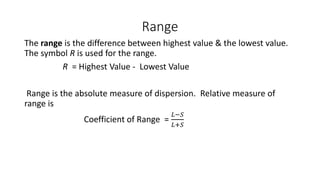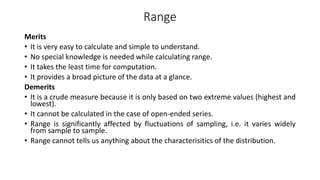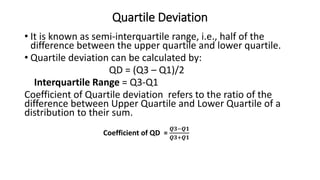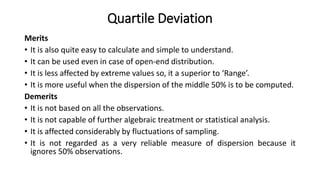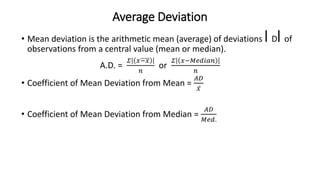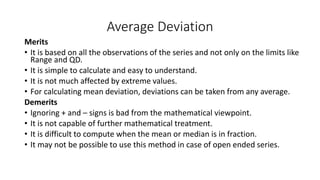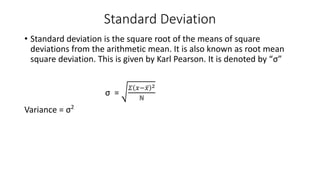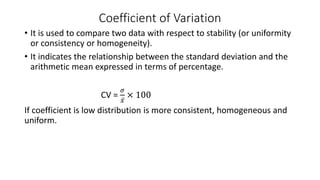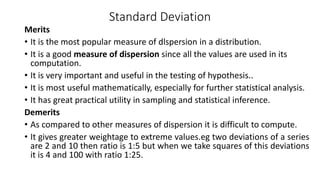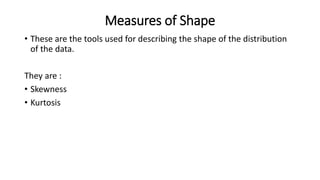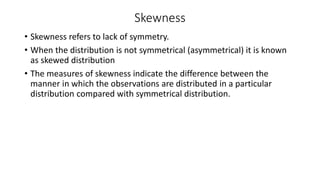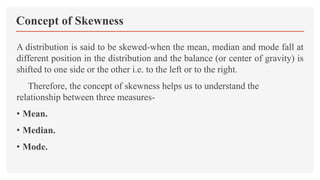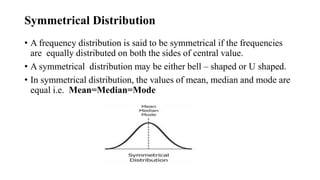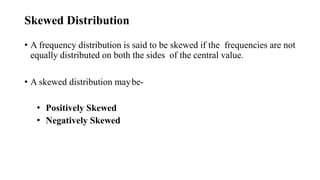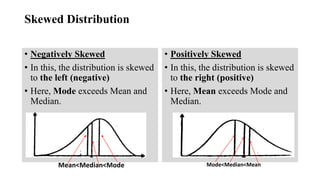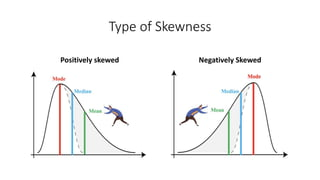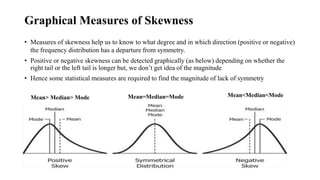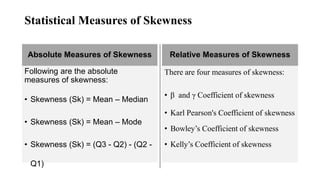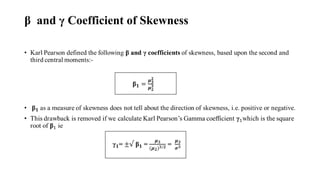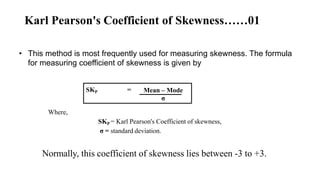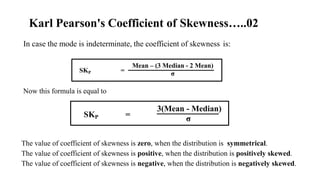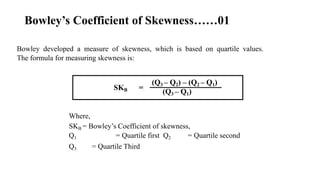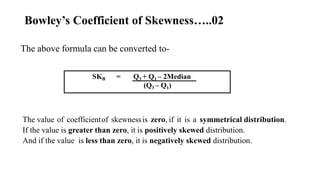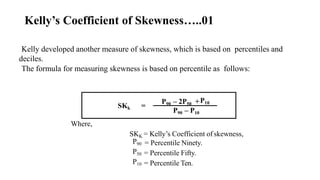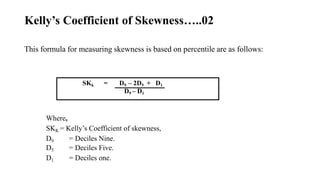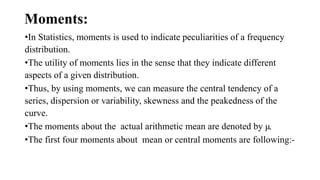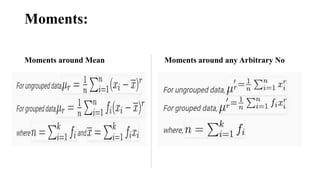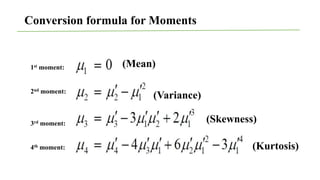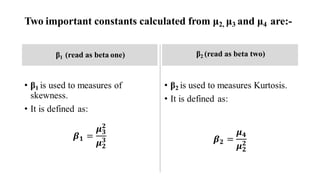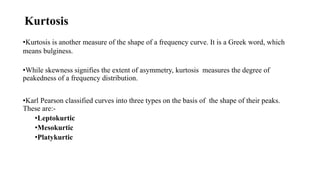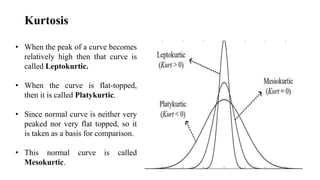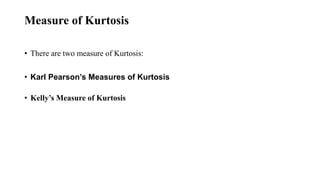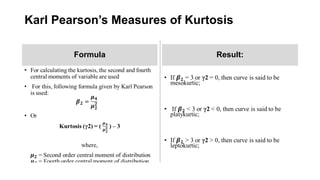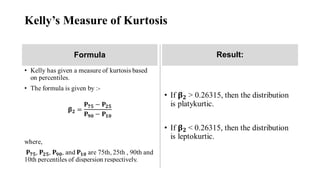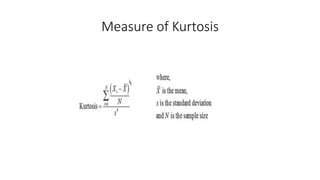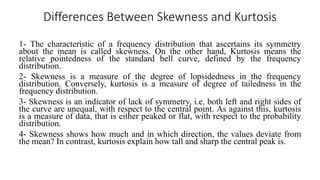1 sur 49

### measures of central tendency.pptx

• 2. Data Description 1. Summarize data, using measures of central tendency, such as the mean, median, mode, and midrange. 2. Describe data, using measures of variation, such as the range, variance, and standard deviation. 3. Identify the position of a data value in a data set, using various measures of position, such as percentiles, deciles, and quartiles. 4. Use the techniques of exploratory data analysis, including boxplots and five-number summaries, to discover various aspects of data.
• 3. Measures of Central Tendency • A central tendency is a single value which is used to represent an entire set of data. • All the data values clustered around central value. • In simple words it is the tendency of the observations(Data Values)to concentrate around a central point. • Statistical measures that indicate the location or position of a central value to describe the central tendency of the entire data are called Measures of Central Tendency. Some important measures of central tendency are: • Mean • Median • Mode • Quartiles • Deciles
• 4. Characteristics of Measures of Central Tendency • It should be easy to understand • It should be easy to compute • It should be based on all the observations • It should be rigidly defined i.e. it must have one and only one interpretation. • It should be capable of further algebraic treatments i.e. it is used for further algebraic computations. • It should have sampling stability i.e. if we take say 10 different samples form the population it will result into almost same measures of central values. • It should not be unduly effected by the presence of extreme values
• 5. Arithmetic Mean • The mean, also known as the arithmetic average, is found by adding the values of the data and dividing by the total number of values. For Grouped Data
• 6. Properties of Arithmetic Mean 1. The sum of the deviations of the items from the arithmetic mean is always zero i.e 𝑥 − 𝑥 = 0 2. The sum of the squares of the deviations of a set of values is minimum when taken from mean. i.e. 𝑥 − 𝑥 2 is minimum. 3. Simple arithmetic means may be combined to give composite mean. 𝑥12 = 𝑁1𝑥1+𝑁2𝑥2 ℕ1+𝑁2
• 7. Median • The median of a distribution is the middle of Central value of the variable when the values are arranged in the order of their magnitude. • It divides the distribution into two equal parts so that half of the data values less than the median while the other half of the values greater than the median. • For ungrouped data: For odd number of observations, Median = [(n + 1)/2]th term. For even number of observations, Median = [(n/2)th term + ((n/2) + 1)th term]/2 • For grouped data: Median = L + [((n/2) - pcf)/f] × i where, L = Lower limit of the median class pcf = Preceding Cumulative frequency to median class i = Class size n = Number of observations Median class = Class where n/2th observation lies
• 8. Mode • The mode is the most commonly occurring value in a distribution. For Grouped data : 𝑀𝑜𝑑𝑒 = 𝐿 + 𝑓1−𝑓0 2𝑓1−𝑓0−𝑓2 𝑥 ⅈ where, L= Lower class interval of Modal class 𝑓1 = Frequency of Modal Class 𝑓0 = Frequency preceding Modal Class 𝑓2 = Frequency Succeeding Modal Class i = Width of class Interval of Modal Class
• 9. Properties & Uses of Central Tendency The Mean 1. The mean is found by using all the values of the data. 2. The mean varies less than the median or mode when samples are taken from the same population and all three measures are computed for these samples. 3. The mean is used in computing other statistics, such as the variance. 4. The mean for the data set is unique and not necessarily one of the data values. 5. The mean cannot be computed for the data in a frequency distribution that has an open-ended class. 6. The mean is affected by extremely high or low values, called outliers, and may not be the appropriate average to use in these situations. The Median 1. The median is used to find the center or middle value of a data set. 2. The median is used when it is necessary to find out whether the data values fall into the upper half or lower half of the distribution. 3. The median is used for an open-ended distribution. 4. The median is affected less than the mean by extremely high or extremely low values. The Mode 1. The mode is used when the most typical case is desired. 2. The mode is the easiest average to compute. 3. The mode can be used when the data are nominal or categorical, such as religious preference, gender, or political affiliation. 4. The mode is not always unique. A data set can have more than one mode, or the mode may not exist for a data set.
• 10. Measures of Dispersion/Variation Dispersion means scatteredness • The degree to which the numerical data tends to spread around an average value is called dispersion or variation of data. • Methods of Studying Dispersion: 1. Range 2. Quartile Deviation 3. Average Deviation 4. Standard Deviation
• 11. Significance of Measuring variation • To determine the reliability of an average : Measures of variations tells whether an average is representative of the entire data or not. If variation is small then we say that average is representative of the entire data. • To serve as a basis for the control of the variability : Variation also determines the nature and cause of variation in order to control the variation itself. It helps in determining the reason behind variation • To compare two or more series with regard to their variability: It also enables to compare two or more series with respect to their variability. The series with less variation is more uniform and consistent. • To facilitate the use of other statistical measures: Variation is also used in other statistical techniques like correlation, testing of hypothesis, production control, cost control etc.
• 12. Properties of Measures Variation • It should be easy to understand • It should be easy to compute • It should be based on all the observations • It should be rigidly defined i.e. it must have one and only one interpretation. • It should be capable of further algebraic treatments i.e. it is used for further algebraic computations. • It should have sampling stability i.e. if we take say 10 different samples form the population it will result into almost same measures of central values. • It should not be unduly effected by the presence of extreme values
• 13. Absolute Vs. Relative Measures of Variations • Absolute Measures of dispersion: Absolute measures of variations are expressed in the same statistical units in which the original data are given like rupees, kilogram, etc. These values are helpful in comparing the variations in two or more distributions which have almost same average value. • Relative Measures of dispersion: Relative measures of dispersion are useful in comparing two sets of data which have different units of measurements. These are expressed as the percentage or the coefficient of the absolute measure of dispersion. Relative measures of variations is the ratio of measure of absolute variation to an Average. It is also called coefficient of variation because coefficient is a pure number that is independent of any unit of measurements
• 14. Range The range is the difference between highest value & the lowest value. The symbol R is used for the range. R = Highest Value - Lowest Value Range is the absolute measure of dispersion. Relative measure of range is Coefficient of Range = 𝐿−𝑆 𝐿+𝑆
• 15. Range Merits • It is very easy to calculate and simple to understand. • No special knowledge is needed while calculating range. • It takes the least time for computation. • It provides a broad picture of the data at a glance. Demerits • It is a crude measure because it is only based on two extreme values (highest and lowest). • It cannot be calculated in the case of open-ended series. • Range is significantly affected by fluctuations of sampling, i.e. it varies widely from sample to sample. • Range cannot tells us anything about the characterisitics of the distribution.
• 16. Quartile Deviation • It is known as semi-interquartile range, i.e., half of the difference between the upper quartile and lower quartile. • Quartile deviation can be calculated by: QD = (Q3 – Q1)/2 Interquartile Range = Q3-Q1 Coefficient of Quartile deviation refers to the ratio of the difference between Upper Quartile and Lower Quartile of a distribution to their sum. Coefficient of QD = 𝑸𝟑−𝑸𝟏 𝑸𝟑+𝑸𝟏
• 17. Quartile Deviation Merits • It is also quite easy to calculate and simple to understand. • It can be used even in case of open-end distribution. • It is less affected by extreme values so, it a superior to ‘Range’. • It is more useful when the dispersion of the middle 50% is to be computed. Demerits • It is not based on all the observations. • It is not capable of further algebraic treatment or statistical analysis. • It is affected considerably by fluctuations of sampling. • It is not regarded as a very reliable measure of dispersion because it ignores 50% observations.
• 18. Average Deviation • Mean deviation is the arithmetic mean (average) of deviations ⎜D⎜of observations from a central value (mean or median). A.D. = 𝛴 𝑥−𝑥 𝑛 or 𝛴 𝑥−𝑀𝑒𝑑𝑖𝑎𝑛 𝑛 • Coefficient of Mean Deviation from Mean = 𝐴𝐷 𝑥 • Coefficient of Mean Deviation from Median = 𝐴𝐷 𝑀𝑒𝑑.
• 19. Average Deviation Merits • It is based on all the observations of the series and not only on the limits like Range and QD. • It is simple to calculate and easy to understand. • It is not much affected by extreme values. • For calculating mean deviation, deviations can be taken from any average. Demerits • Ignoring + and – signs is bad from the mathematical viewpoint. • It is not capable of further mathematical treatment. • It is difficult to compute when the mean or median is in fraction. • It may not be possible to use this method in case of open ended series.
• 20. Standard Deviation • Standard deviation is the square root of the means of square deviations from the arithmetic mean. It is also known as root mean square deviation. This is given by Karl Pearson. It is denoted by “σ” σ = 𝛴 𝑥−𝑥 2 ℕ Variance = σ2
• 21. Coefficient of Variation • It is used to compare two data with respect to stability (or uniformity or consistency or homogeneity). • It indicates the relationship between the standard deviation and the arithmetic mean expressed in terms of percentage. CV = 𝜎 𝑥 × 100 If coefficient is low distribution is more consistent, homogeneous and uniform.
• 22. Standard Deviation Merits • It is the most popular measure of dlspersion in a distribution. • It is a good measure of dispersion since all the values are used in its computation. • It is very important and useful in the testing of hypothesis.. • It is most useful mathematically, especially for further statistical analysis. • It has great practical utility in sampling and statistical inference. Demerits • As compared to other measures of dispersion it is difficult to compute. • It gives greater weightage to extreme values.eg two deviations of a series are 2 and 10 then ratio is 1:5 but when we take squares of this deviations it is 4 and 100 with ratio 1:25.
• 23. Measures of Shape • These are the tools used for describing the shape of the distribution of the data. They are : • Skewness • Kurtosis
• 24. Skewness • Skewness refers to lack of symmetry. • When the distribution is not symmetrical (asymmetrical) it is known as skewed distribution • The measures of skewness indicate the difference between the manner in which the observations are distributed in a particular distribution compared with symmetrical distribution.
• 25. Concept of Skewness A distribution is said to be skewed-when the mean, median and mode fall at different position in the distribution and the balance (or center of gravity) is shifted to one side or the other i.e. to the left or to the right. Therefore, the concept of skewness helps us to understand the relationship between three measures- • Mean. • Median. • Mode.
• 26. Symmetrical Distribution • A frequency distribution is said to be symmetrical if the frequencies are equally distributed on both the sides of central value. • A symmetrical distribution may be either bell – shaped or U shaped. • In symmetrical distribution, the values of mean, median and mode are equal i.e. Mean=Median=Mode
• 27. Skewed Distribution • A frequency distribution is said to be skewed if the frequencies are not equally distributed on both the sides of the central value. • A skewed distribution maybe- • Positively Skewed • Negatively Skewed
• 28. Skewed Distribution • Negatively Skewed • In this, the distribution is skewed to the left (negative) • Here, Mode exceeds Mean and Median. • Positively Skewed • In this, the distribution is skewed to the right (positive) • Here, Mean exceeds Mode and Median. Mean<Median<Mode Mode<Median<Mean
• 29. Type of Skewness Positively skewed Negatively Skewed
• 30. Graphical Measures of Skewness • Measures of skewness help us to know to what degree and in which direction (positive or negative) the frequency distribution has a departure from symmetry. • Positive or negative skewness can be detected graphically (as below) depending on whether the right tail or the left tail is longer but, we don’t get idea of the magnitude • Hence some statistical measures are required to find the magnitude of lack of symmetry Mean=Median=Mode Mean<Median<Mode Mean> Median> Mode Symmetrical Skewed to the Left Skewed to the Right
• 31. Statistical Measures of Skewness Absolute Measures of Skewness Following are the absolute measures of skewness: • Skewness (Sk) = Mean – Median • Skewness (Sk) = Mean – Mode • Skewness (Sk) = (Q3 - Q2) - (Q2 - Q1) Relative Measures of Skewness There are four measures of skewness: • β and γ Coefficient of skewness • Karl Pearson's Coefficient of skewness • Bowley’s Coefficient of skewness • Kelly’s Coefficient of skewness
• 32. β and γ Coefficient of Skewness
• 33. Karl Pearson's Coefficient of Skewness……01 • This method is most frequently used for measuring skewness. The formula for measuring coefficient of skewness is given by Where, SKP = Karl Pearson's Coefficient of skewness, σ = standard deviation. SKP = Mean – Mode σ Normally, this coefficient of skewness lies between -3 to +3.
• 34. In case the mode is indeterminate, the coefficient of skewness is: Now this formula is equal to The value of coefficient of skewness is zero, when the distribution is symmetrical. The value of coefficient of skewness is positive, when the distribution is positively skewed. The value of coefficient of skewness is negative, when the distribution is negatively skewed. SKP = Mean – (3 Median - 2 Mean) σ SKP = 3(Mean - Median) σ Karl Pearson's Coefficient of Skewness…..02
• 35. Bowley’s Coefficient of Skewness……01 Bowley developed a measure of skewness, which is based on quartile values. The formula for measuring skewness is: Where, SKB = Bowley’s Coefficient of skewness, Q1 = Quartile first Q2 = Quartile second Q3 = Quartile Third SKB = (Q3 – Q2) – (Q2 – Q1) (Q3 – Q1)
• 36. Bowley’s Coefficient of Skewness…..02 The above formula can be converted to- The value of coefficientof skewnessis zero, if it is a symmetrical distribution. If the value is greater than zero, it is positively skewed distribution. And if the value is less than zero, it is negatively skewed distribution. SKB = Q3 + Q1 – 2Median (Q3 – Q1)
• 37. Kelly’s Coefficient of Skewness…..01 Kelly developed another measure of skewness, which is based on percentiles and deciles. The formula for measuring skewness is based on percentile as follows: Where, SKK = Kelly’s Coefficient of skewness, P90 P50 P10 = Percentile Ninety. = Percentile Fifty. = Percentile Ten. SKk = P10 P90 – 2P50 + P90 – P10
• 38. Kelly’s Coefficient of Skewness…..02 This formula for measuring skewness is based on percentile are as follows: Where, SKK = Kelly’s Coefficient of skewness, D9 = Deciles Nine. D5 = Deciles Five. D1 = Deciles one. SKk = D9 – 2D5 + D1 D9 – D1
• 39. Moments: •In Statistics, moments is used to indicate peculiarities of a frequency distribution. •The utility of moments lies in the sense that they indicate different aspects of a given distribution. •Thus, by using moments, we can measure the central tendency of a series, dispersion or variability, skewness and the peakedness of the curve. •The moments about the actual arithmetic mean are denoted by μ. •The first four moments about mean or central moments are following:-
• 40. Moments: Moments around Mean Moments around any Arbitrary No
• 41. Conversion formula for Moments (Mean) (Variance) (Skewness) (Kurtosis) 1st moment: 2nd moment: 3rd moment: 4th moment:
• 42. Two important constants calculated from μ2, μ3 and μ4 are:- β1 (read as beta one) β2 (read as beta two)
• 43. Kurtosis •Kurtosis is another measure of the shape of a frequency curve. It is a Greek word, which means bulginess. •While skewness signifies the extent of asymmetry, kurtosis measures the degree of peakedness of a frequency distribution. •Karl Pearson classified curves into three types on the basis of the shape of their peaks. These are:- •Leptokurtic •Mesokurtic •Platykurtic
• 44. Kurtosis • When the peak of a curve becomes relatively high then that curve is called Leptokurtic. • When the curve is flat-topped, then it is called Platykurtic. • Since normal curve is neither very peaked nor very flat topped, so it is taken as a basis for comparison. • This normal curve is called Mesokurtic.
• 45. Measure of Kurtosis • There are two measure of Kurtosis: • Karl Pearson’s Measures of Kurtosis • Kelly’s Measure of Kurtosis
• 46. Karl Pearson’s Measures of Kurtosis Formula Result:
• 47. Kelly’s Measure of Kurtosis Formula Result:
• 49. Differences Between Skewness and Kurtosis 1- The characteristic of a frequency distribution that ascertains its symmetry about the mean is called skewness. On the other hand, Kurtosis means the relative pointedness of the standard bell curve, defined by the frequency distribution. 2- Skewness is a measure of the degree of lopsidedness in the frequency distribution. Conversely, kurtosis is a measure of degree of tailedness in the frequency distribution. 3- Skewness is an indicator of lack of symmetry, i.e. both left and right sides of the curve are unequal, with respect to the central point. As against this, kurtosis is a measure of data, that is either peaked or flat, with respect to the probability distribution. 4- Skewness shows how much and in which direction, the values deviate from the mean? In contrast, kurtosis explain how tall and sharp the central peak is.

### Notes de l'éditeur

1. Mean = 64; Median =64.8 and Mode= 65.2....... Negatively Skewed Mean>Median>Mode.... Positively skewed
2. Mode = 3 Median – 2 Mean
3. If Sk = + or – 3: Perfectly Positively/Negatively Skewed. If Sk = +/- 2 to 2.99 : High degree Positive/Negative skewness If Sk = +/- 1 to 1.99 : Moderate degree Positive/Negative skewness; If Sk = +/- 0.1 to 0.99 : Low degree Positive/Negative skewness